# Paul Apivat Data Journey# Mining Thai Food Text with R

This is Part 3 in the Thai Food Dishes series. See Part 1 and Part 2.

### Text Mining

One way to answer this question is to use text mining to tokenize by either word and count the words by frequency as one measure of popularity.

In the below bar chart, we see frequency of words across all Thai Dishes. Mu (หมู) which means pork in Thai appears most frequently across all dish types and sub-grouping. Next we have kaeng (แกง) which means curry. Phat (ผัด) comings in third suggesting "stir-fry" is a popular cooking mode.

As we can see not all words refer to raw materials, so we may not be able to answer this question directly.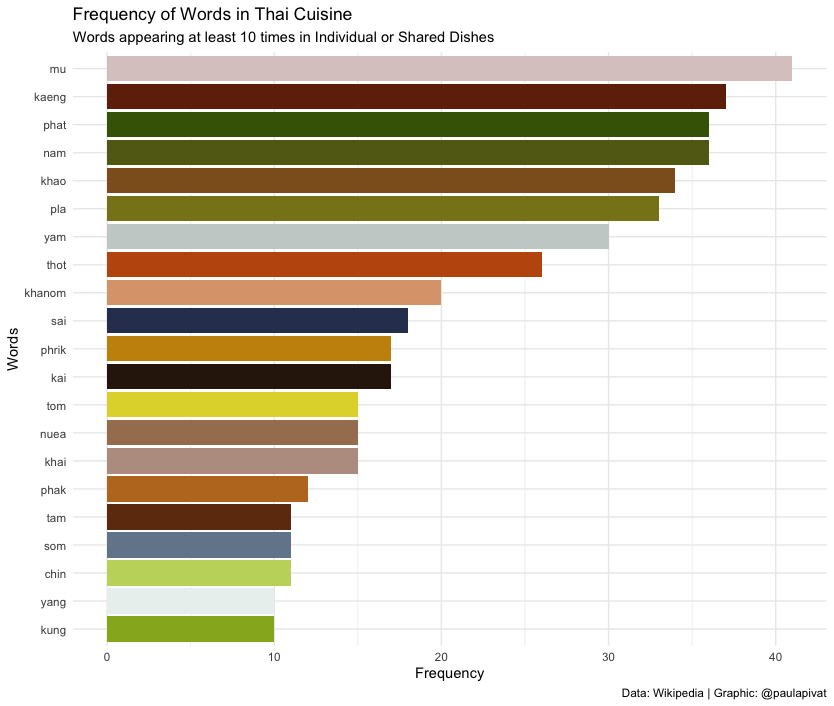``````library(tidytext)
library(scales)

# new csv file after data cleaning (see above)

df %>%
select(Thai_name, Thai_script) %>%
# can substitute 'word' for ngrams, sentences, lines
unnest_tokens(ngrams, Thai_name) %>%
# to reference thai spelling: group_by(Thai_script)
group_by(ngrams) %>%
tally(sort = TRUE) %>%  # alt: count(sort = TRUE)
filter(n > 9) %>%
# visualize
# pipe directly into ggplot2, because using tidytools
ggplot(aes(x = n, y = reorder(ngrams, n))) +
geom_col(aes(fill = ngrams)) +
scale_fill_manual(values = c(
"#c3d66b",
"#70290a",
"#2f1c0b",
"#ba9d8f",
"#dda37b",
"#8f5e23",
"#96b224",
"#dbcac9",
"#626817",
"#a67e5f",
"#be7825",
"#446206",
"#c8910b",
"#88821b",
"#313d5f",
"#73869a",
"#6f370f",
"#c0580d",
"#e0d639",
"#c9d0ce",
"#ebf1f0",
"#50607b"
)) +
theme_minimal() +
theme(legend.position = "none") +
labs(
x = "Frequency",
y = "Words",
title = "Frequency of Words in Thai Cuisine",
subtitle = "Words appearing at least 10 times in Individual or Shared Dishes",
caption = "Data: Wikipedia | Graphic: @paulapivat"
)
``````

We can also see words common to both Individual and Shared Dishes. We see other words like nuea (beef), phrik (chili) and kaphrao (basil leaves).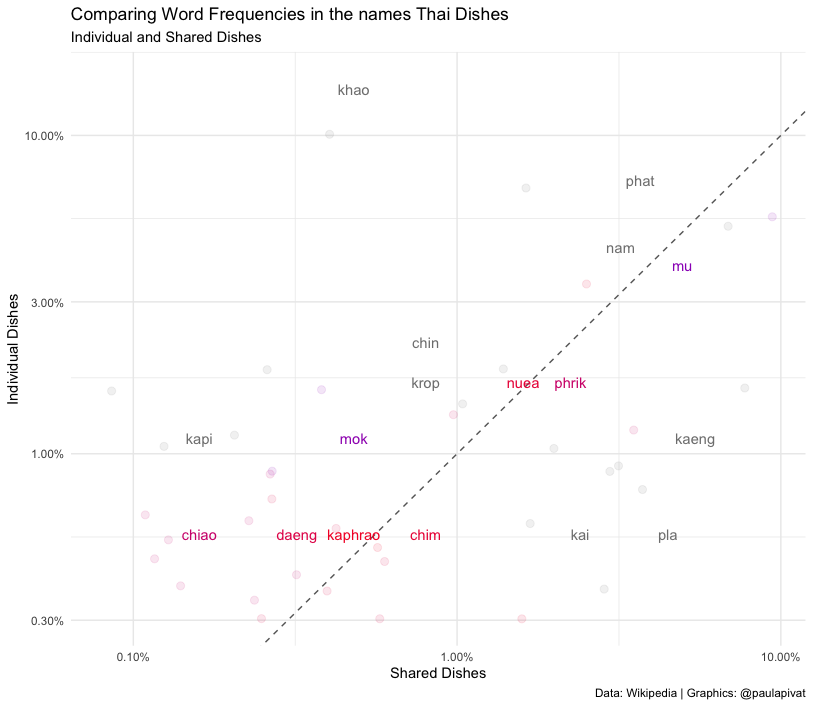``````# frequency for Thai_dishes (Major Grouping) ----

# comparing Individual and Shared Dishes (Major Grouping)
thai_name_freq <- df %>%
select(Thai_name, Thai_script, major_grouping) %>%
unnest_tokens(ngrams, Thai_name) %>%
count(ngrams, major_grouping) %>%
group_by(major_grouping) %>%
mutate(proportion = n / sum(n)) %>%
select(major_grouping, ngrams, proportion) %>%
gather(major_grouping, proportion, c(`Shared dishes`)) %>%
select(ngrams, `Individual dishes`, major_grouping, proportion)

# Expect warming message about missing values
ggplot(thai_name_freq, aes(x = proportion, y = `Individual dishes`,
color = abs(`Individual dishes` - proportion))) +
geom_abline(color = 'gray40', lty = 2) +
geom_jitter(alpha = 0.1, size = 2.5, width = 0.3, height = 0.3) +
geom_text(aes(label = ngrams), check_overlap = TRUE, vjust = 1.5) +
scale_x_log10(labels = percent_format()) +
scale_y_log10(labels = percent_format()) +
low = "red", high = "blue") +    # low = "darkslategray4", high = "gray75"
theme_minimal() +
theme(legend.position = "none",
legend.text = element_text(angle = 45, hjust = 1)) +
labs(y = "Individual Dishes",
x = "Shared Dishes",
color = NULL,
title = "Comparing Word Frequencies in the names Thai Dishes",
subtitle = "Individual and Shared Dishes",
caption = "Data: Wikipedia | Graphics: @paulapivat")
``````

#### Which raw materials are most important?

We can only learn so much from frequency, so text mining practitioners have created term frequency - inverse document frequency to better reflect how important a word is in a document or corpus (further details here).

Again, the words don't necessarily refer to raw materials, so this question can't be fully answered directly here.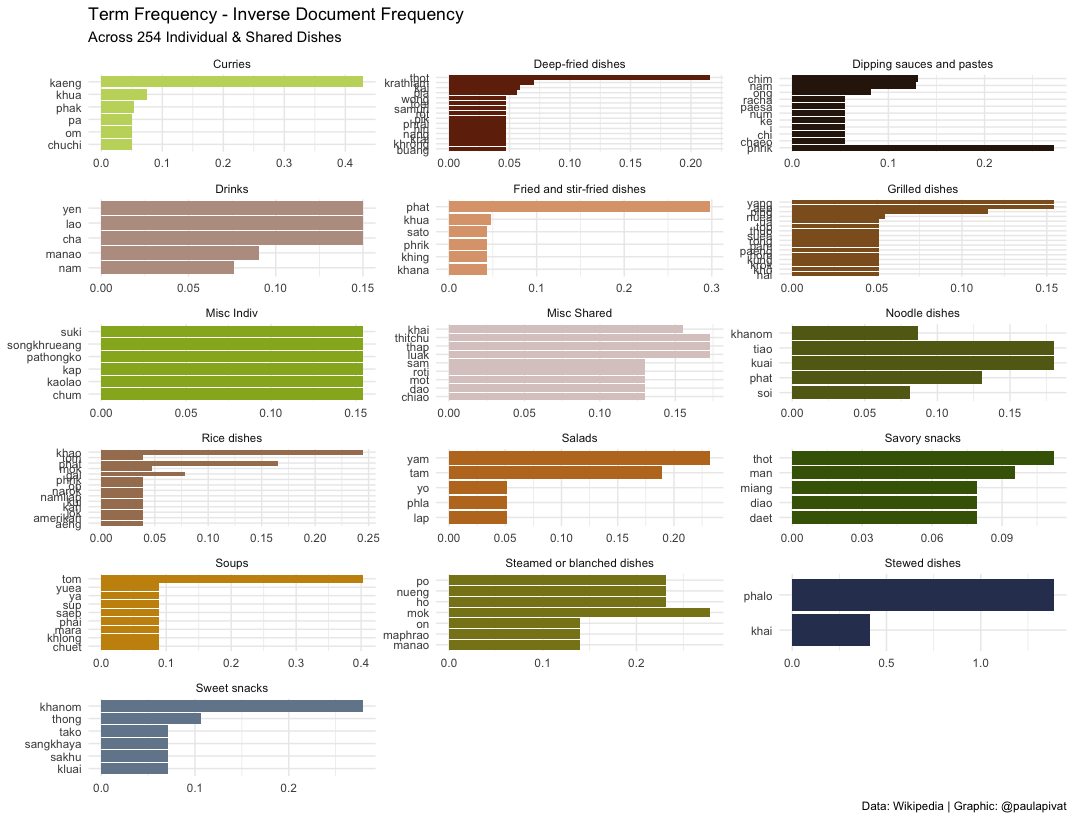#### Could you learn about Thai food just from the names of the dishes?

We learned just from frequency and "term frequency - inverse document frequency" not only the most frequent words, but the relative importance within the current set of words that we have tokenized with `tidytext`. This informs us of not only popular raw materials (Pork), but also dish types (Curries) and other popular mode of preparation (Stir-Fry).

We can even examine the network of relationships between words. Darker arrows suggest a stronger relationship between pairs of words, for example "nam phrik" is a strong pairing. This means "chili sauce" in Thai and suggests the important role that it plays across many types of dishes.

We learned above that "mu" (pork) appears frequently. Now we see that "mu" and "krop" are more related than other pairings (note: "mu krop" means "crispy pork"). We also saw above that "khao" appears frequently in Rice dishes. This alone is not surprising as "khao" means rice in Thai, but we see here "khao phat" is strongly related suggesting that fried rice ("khao phat") is quite popular.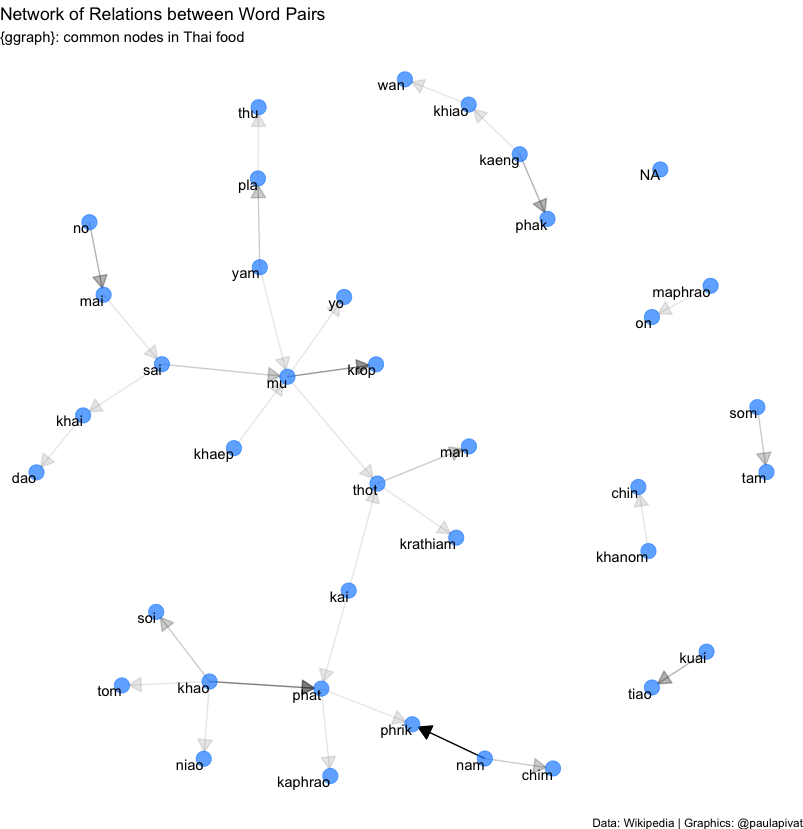``````# Visualizing a network of Bi-grams with {ggraph} ----
library(igraph)
library(ggraph)
set.seed(2021)

thai_dish_bigram_counts <- df %>%
select(Thai_name, minor_grouping) %>%
unnest_tokens(bigram, Thai_name, token = "ngrams", n = 2) %>%
separate(bigram, c("word1", "word2"), sep = " ") %>%
count(word1, word2, sort = TRUE)

# filter for relatively common combinations (n > 2)
thai_dish_bigram_graph <- thai_dish_bigram_counts %>%
filter(n > 2) %>%
graph_from_data_frame()

# polishing operations to make a better looking graph
a <- grid::arrow(type = "closed", length = unit(.15, "inches"))

set.seed(2021)
ggraph(thai_dish_bigram_graph, layout = "fr") +
geom_edge_link(aes(edge_alpha = n), show.legend = FALSE,
arrow = a, end_cap = circle(.07, 'inches')) +
geom_node_point(color = "dodgerblue", size = 5, alpha = 0.7) +
geom_node_text(aes(label = name), vjust = 1, hjust = 1) +
labs(
title = "Network of Relations between Word Pairs",
subtitle = "{ggraph}: common nodes in Thai food",
caption = "Data: Wikipedia | Graphics: @paulapivat"
) +
theme_void()
``````

Finally, we may be interested in word relationships within individual dishes.

The below graph shows a network of word pairs with moderate-to-high correlations. We can see certain words clustered near each other with relatively dark lines: kaeng (curry), pet (spicy), wan (sweet), khiao (green curry), phrik (chili) and mu (pork). These words represent a collection of ingredient, mode of cooking and description that are generally combined.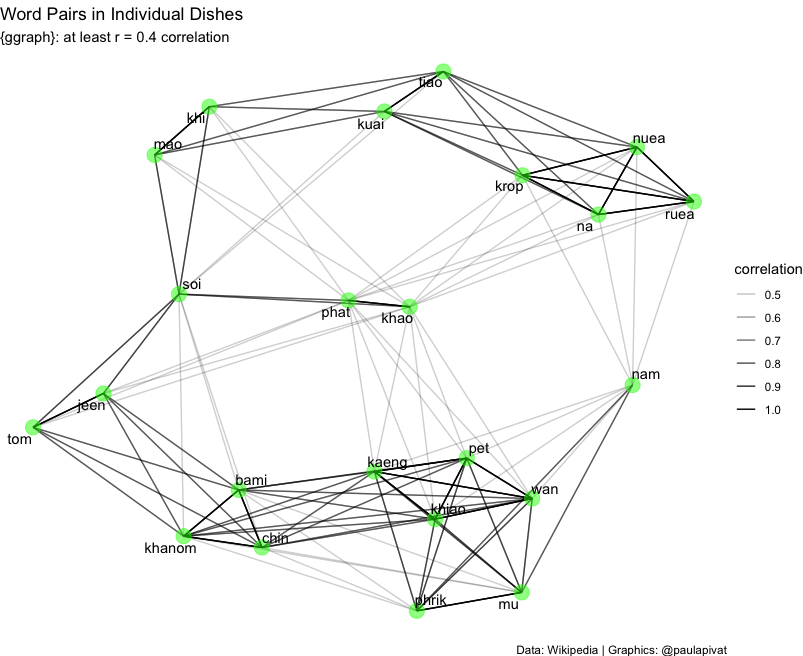``````set.seed(2021)

# Individual Dishes
individual_dish_words <- df %>%
select(major_grouping, Thai_name) %>%
filter(major_grouping == 'Individual dishes') %>%
mutate(section = row_number() %/% 10) %>%
filter(section > 0) %>%
unnest_tokens(word, Thai_name)  # assume no stop words

individual_dish_cors <- individual_dish_words %>%
group_by(word) %>%
filter(n() >= 2) %>%     # looking for co-occuring words, so must be 2 or greater
pairwise_cor(word, section, sort = TRUE)

individual_dish_cors %>%
filter(correlation < -0.40) %>%
graph_from_data_frame() %>%
ggraph(layout = "fr") +
geom_edge_link(aes(edge_alpha = correlation, size = correlation), show.legend = TRUE) +
geom_node_point(color = "green", size = 5, alpha = 0.5) +
geom_node_text(aes(label = name), repel = TRUE) +
labs(
title = "Word Pairs in Individual Dishes",
subtitle = "{ggraph}: Negatively correlated (r = -0.4)",
caption = "Data: Wikipedia | Graphics: @paulapivat"
) +
theme_void()
``````

#### Summary

We have completed an exploratory data project where we scraped, clean, manipulated and visualized data using a combination of Python and R. We also used the `tidytext` package for basic text mining task to see if we could gain some insights into Thai cuisine using words from dish names scraped off Wikipedia.

For more content on data science, R, Python, SQL and more, find me on Twitter.